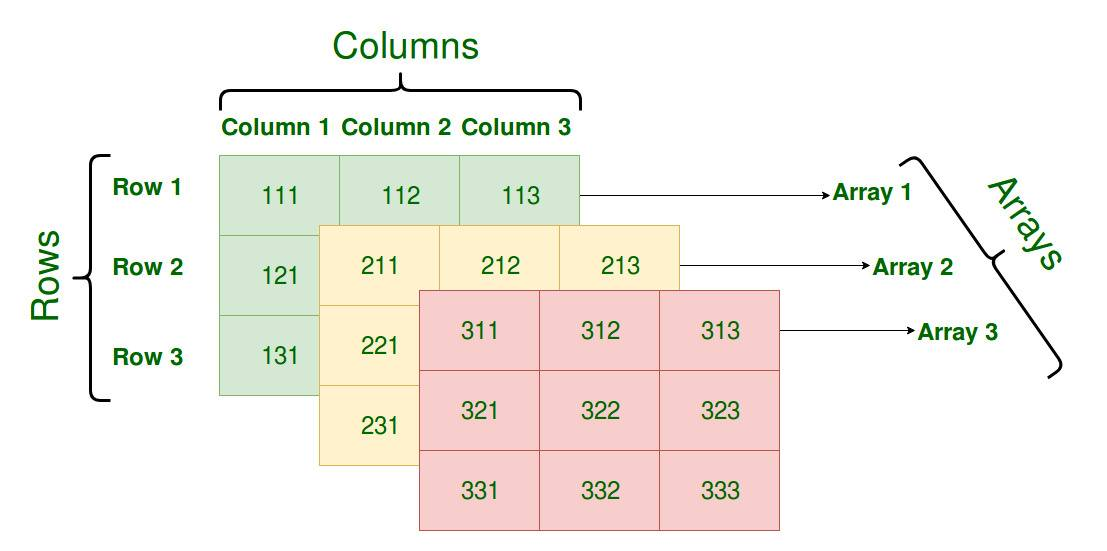Go 语言数组的实现原理

# 3.1 数组 #

## 3.1.1 概述 #int
interface{}


Go 语言数组在初始化之后大小就无法改变，存储元素类型相同、但是大小不同的数组类型在 Go 语言看来也是完全不同的，只有两个条件都相同才是同一类型。

func NewArray(elem *Type, bound int64) *Type {
if bound < 0 {
Fatalf("NewArray: invalid bound %v", bound)
}
t := New(TARRAY)
t.Extra = &Array{Elem: elem, Bound: bound}
t.SetNotInHeap(elem.NotInHeap())
return t
}


## 3.1.2 初始化 #

Go 语言的数组有两种不同的创建方式，一种是显式的指定数组大小，另一种是使用 [...]T 声明数组，Go 语言会在编译期间通过源代码推导数组的大小：

arr1 := int{1, 2, 3}
arr2 := [...]int{1, 2, 3}


### 上限推导 #

func typecheckcomplit(n *Node) (res *Node) {
...
if n.Right.Op == OTARRAY && n.Right.Left != nil && n.Right.Left.Op == ODDD {
n.Right.Right = typecheck(n.Right.Right, ctxType)
if n.Right.Right.Type == nil {
n.Type = nil
return n
}
elemType := n.Right.Right.Type

length := typecheckarraylit(elemType, -1, n.List.Slice(), "array literal")

n.Op = OARRAYLIT
n.Type = types.NewArray(elemType, length)
n.Right = nil
return n
}
...

switch t.Etype {
case TARRAY:
typecheckarraylit(t.Elem(), t.NumElem(), n.List.Slice(), "array literal")
n.Op = OARRAYLIT
n.Right = nil
}
}


### 语句转换 #

1. 当元素数量小于或者等于 4 个时，会直接将数组中的元素放置在栈上；
2. 当元素数量大于 4 个时，会将数组中的元素放置到静态区并在运行时取出；
func anylit(n *Node, var_ *Node, init *Nodes) {
t := n.Type
switch n.Op {
case OSTRUCTLIT, OARRAYLIT:
if n.List.Len() > 4 {
...
}

fixedlit(inInitFunction, initKindLocalCode, n, var_, init)
...
}
}


func fixedlit(ctxt initContext, kind initKind, n *Node, var_ *Node, init *Nodes) {
var splitnode func(*Node) (a *Node, value *Node)
...

for _, r := range n.List.Slice() {
a, value := splitnode(r)
a = nod(OAS, a, value)
a = typecheck(a, ctxStmt)
switch kind {
case initKindStatic:
genAsStatic(a)
case initKindLocalCode:
a = orderStmtInPlace(a, map[string][]*Node{})
a = walkstmt(a)
init.Append(a)
}
}
}


var arr int
arr = 1
arr = 2
arr = 3


func anylit(n *Node, var_ *Node, init *Nodes) {
t := n.Type
switch n.Op {
case OSTRUCTLIT, OARRAYLIT:
if n.List.Len() > 4 {
vstat := staticname(t)

fixedlit(inNonInitFunction, initKindStatic, n, vstat, init)

a := nod(OAS, var_, vstat)
a = typecheck(a, ctxStmt)
a = walkexpr(a, init)
init.Append(a)
break
}

...
}
}


var arr int
statictmp_0 = 1
statictmp_0 = 2
statictmp_0 = 3
statictmp_0 = 4
statictmp_0 = 5
arr = statictmp_0


## 3.1.3 访问和赋值 #func typecheck1(n *Node, top int) (res *Node) {
switch n.Op {
case OINDEX:
ok |= ctxExpr
l := n.Left  // array
r := n.Right // index
switch n.Left.Type.Etype {
case TSTRING, TARRAY, TSLICE:
...
if n.Right.Type != nil && !n.Right.Type.IsInteger() {
yyerror("non-integer array index %v", n.Right)
break
}
if !n.Bounded() && Isconst(n.Right, CTINT) {
x := n.Right.Int64()
if x < 0 {
yyerror("invalid array index %v (index must be non-negative)", n.Right)
} else if n.Left.Type.IsArray() && x >= n.Left.Type.NumElem() {
yyerror("invalid array index %v (out of bounds for %d-element array)", n.Right, n.Left.Type.NumElem())
}
}
}
...
}
}

1. 访问数组的索引是非整数时，报错 “non-integer array index %v”；
2. 访问数组的索引是负数时，报错 “invalid array index %v (index must be non-negative)"；
3. 访问数组的索引越界时，报错 “invalid array index %v (out of bounds for %d-element array)"；

arr: invalid array index 4 (out of bounds for 3-element array)
arr[i]: panic: runtime error: index out of range  with length 3


Go 语言运行时在发现数组、切片和字符串的越界操作会由运行时的 runtime.panicIndexruntime.goPanicIndex 触发程序的运行时错误并导致崩溃退出：

TEXT runtime·panicIndex(SB),NOSPLIT,$0-8 MOVL AX, x+0(FP) MOVL CX, y+4(FP) JMP runtime·goPanicIndex(SB) func goPanicIndex(x int, y int) { panicCheck1(getcallerpc(), "index out of range") panic(boundsError{x: int64(x), signed: true, y: y, code: boundsIndex}) }  当数组的访问操作 OINDEX 成功通过编译器的检查后，会被转换成几个 SSA 指令，假设我们有如下所示的 Go 语言代码，通过如下的方式进行编译会得到 ssa.html 文件： package check func outOfRange() int { arr := int{1, 2, 3} i := 4 elem := arr[i] return elem }$ GOSSAFUNC=outOfRange go build array.go
dumped SSA to ./ssa.html


start 阶段生成的 SSA 代码就是优化之前的第一版中间代码，下面展示的部分是 elem := arr[i] 对应的中间代码，在这段中间代码中我们发现 Go 语言为数组的访问操作生成了判断数组上限的指令 IsInBounds 以及当条件不满足时触发程序崩溃的 PanicBounds 指令：

b1:
...
v22 (6) = LocalAddr <*int> {arr} v2 v20
v23 (6) = IsInBounds <bool> v21 v11
If v23 → b2 b3 (likely) (6)

b2: ← b1-
v26 (6) = PtrIndex <*int> v22 v21
v27 (6) = Copy <mem> v20
v28 (6) = Load <int> v26 v27 (elem[int])
...
Ret v30 (+7)

b3: ← b1-
v24 (6) = Copy <mem> v20
v25 (6) = PanicBounds <mem>  v21 v11 v24
Exit v25 (6)


b1:
...
v21 (5) = LocalAddr <*int> {arr} v2 v20
v22 (5) = PtrIndex <*int> v21 v14
v23 (5) = Load <int> v22 v20 (elem[int])
...


Go 语言对于数组的访问还是有着比较多的检查的，它不仅会在编译期间提前发现一些简单的越界错误并插入用于检测数组上限的函数调用，还会在运行期间通过插入的函数保证不会发生越界。

b1:
...
v21 (5) = LocalAddr <*int> {arr} v2 v19
v22 (5) = PtrIndex <*int> v21 v13
v23 (5) = Store <mem> {int} v22 v20 v19
...


## 3.1.5 延伸阅读 #

1. Array data structure, https://en.wikipedia.org/wiki/Array_data_structure ↩︎

## 购买纸质书